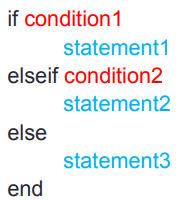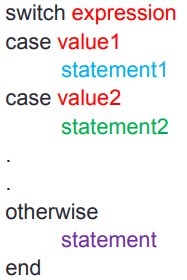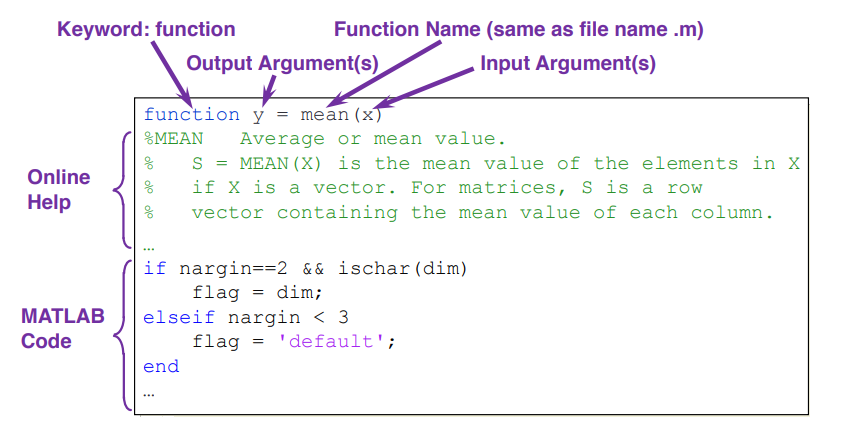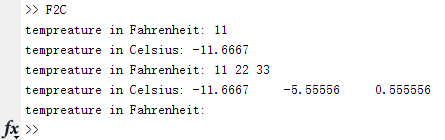# MATLAB02:结构化编程和函数定义

11 篇文章 467 订阅

pdf版本笔记的下载地址: MATLAB02_结构化编程和函数定义（访问密码：3834）

# 结构化编程

## 流程控制语句和逻辑运算符

if, elseif, elseif语句为真,则执行子句
switch, case, otherwise根据switch语句内容判断执行哪个子句
while重复执行子句直到while中的条件为假
for执行子句固定次数
try, catch执行子句并捕获执行过程中的异常
break跳出循环
continue直接进入下一次循环
end结束子句
pause暂停程序
return返回到调用函数处

MATLAB还有以下逻辑运算符:

<小于
<=小于或等于
>大于
>=大于或等于
==等于
~=不等于
&&
||

&&||运算符支持逻辑短路功能.

### 流程控制语句示例

1. if语句:if rem(a, 2) == 0
disp('a is even');
else
disp('a is odd');
end

2. switch语句:switch input_num
case -1
disp('negative 1');
case 0
disp('zero');
case 1
disp('positive 1');
otherwise
disp('other value');
end

3. while语句:n = 1;
while prod(1:n) < 1e100
n = n + 1;
end

4. for语句:for n=1:10
a(n)=2^n;
end
disp(a)

5. break语句:

x = 2; k = 0; error = inf;
error_threshold = 1e-32;
while error > error_threshold
if k > 100
break
end
x = x - sin(x)/cos(x);
error = abs(x - pi);
k = k + 1;
end


### 使用循环语句应尽量预先分配内存空间

• 程序一:

tic
for ii = 1:2000
for jj = 1:2000
A(ii,jj) = ii + jj;
end
end
toc


程序输出Elapsed time is 4.616199 seconds.

• 程序二:

tic
A = zeros(2000, 2000);		% 预先为变量分配内存空间
for ii = 1:size(A,1)
for jj = 1:size(A,2)
A(ii,jj) = ii + jj;
end
end
toc


程序输出Elapsed time is 2.786401 seconds.

## 编写脚本时应注意的问题

### 在脚本开头应添加语句清空工作区

clear all	% 清空工作区内存中的变量
close all 	% 关闭之前程序绘制的图像
clc			% 清空之前程序在终端的输出


### 使用省略号...拼接多行语句

annPoints_sampled = annPoints(annPoints(:,1)>x1 & ...
annPoints(:,1) < x2 & ...
annPoints(:,2) > y1 & ...
annPoints(:,2) < y2);


# 函数

## 查看内置函数

edit(which('mean.m'))## 以函数名.m文件形式定义函数

function [输出变量名] = 函数名(输入变量名)
% 函数的文档


• function是一个关键字,声明该文件中保存的是一个函数.
• 输入变量输出变量是非必须的,函数既可以没有输入变量,也可以没有输出变量.
• 函数名应与.m文件名相同,且不包含特殊字符(最好不要有中文).

### MATLAB内置的函数参数

imputname输入变量名列表
mfilename函数源代码文件名
nargin输入变量数
nargout输出变量个数
varargin可变长输入参数列表
varargout可变长输出参数列表

MATLAB不提供其他高级语言的指定默认参数值以及函数重载等语法,但灵活使用上述内置的函数参数,可以在一定程度上实现指定默认参数值以及方法重载:

function [volume]=pillar(Do,Di,height)
if nargin==2,
height=1;
end
volume=abs(Do.^2-Di.^2).*height*pi/4;


### MATLAB函数定义示例1

x = x 0 + v 0 t + 1 2 g t 2 x = x_0 + v_0 t + \frac{1}{2} g t^2

function x = freebody(x0,v0,t)
% calculation of free falling
% x0: initial displacement in m
% v0: initial velocity in m/sec
% t: the elapsed time in sec
% x: the depth of falling in m
x = x0 + v0.*t + 1/2*9.8*t.*t;


freebody(0, 0, 2)			% 得到 19.6000
freebody(0, 0, [0 1 2 3])	% 得到 [0 4.9000 19.6000 44.1000]
freebody(0, 0, [0 1; 2 3])	% 得到 [0 4.9000; 19.6000 44.1000]


### MATLAB函数定义示例2

function F2C()
while 1
F_degree = input('tempreature in Fahrenheit: ', 's');
F_degree = str2num(F_degree);
if isempty(F_degree)
return
end
C_degree = (F_degree-32)*5/9;
disp(['tempreature in Celsius: ' num2str(C_degree)])
end(需要按Ctrl+C退出程序)

## 以函数句柄形式定义函数

函数句柄 = @(输入变量) 输出变量


f = @(x) exp(-2*x);
x = 0:0.1:2;
plot(x, f(x));


pdf版本笔记的下载地址: MATLAB02_结构化编程和函数定义（访问密码：3834）

01-19
03-3097万+06-281万+
01-20125
01-2270
03-1747
07-23137
04-25123
08-07147
09-01456
07-24
06-041349
08-179510
09-20
06-03
01-22438点击重新获取扫码支付余额充值